### Home > PC > Chapter 6 > Lesson 6.1.1 > Problem6-16

6-16.
1. The amount of water evaporating from a conical container varies directly with the exposed surface area (circular region) of the water in the cone. The height of the cone is 10 meters when full. When the height of the water is 5 meters, the radius of the exposed circular region is 3 meters. At this point, water is evaporating at a rate of 0.2 liters per hour. Homework Help ✎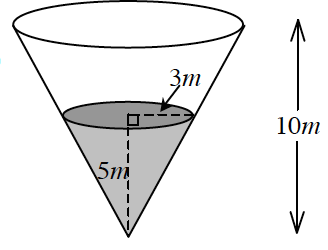1. How fast is the water evaporating when the container is full?

2. How fast is the water evaporating when it is half full? (Reminder V =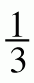πr 2h.)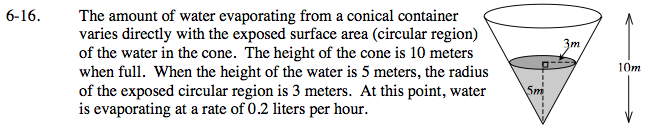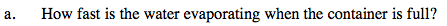Determine the radius when the cone is filled.

$\frac{3}{5}=\frac{r}{10}$

Set up and solve a proportion relating the area with the rate of evaporation.

$\frac{9\pi}{0.2}=\frac{36\pi }{x}$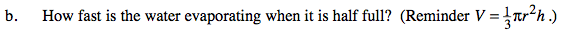When the cone is full, it has 120π m3 of water in it.
What is the volume of water in the cone when it is half full?

Calculate r when the cone is half full. Then set up a proportion with area and evaporation rate similar to the one in part (a).